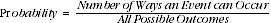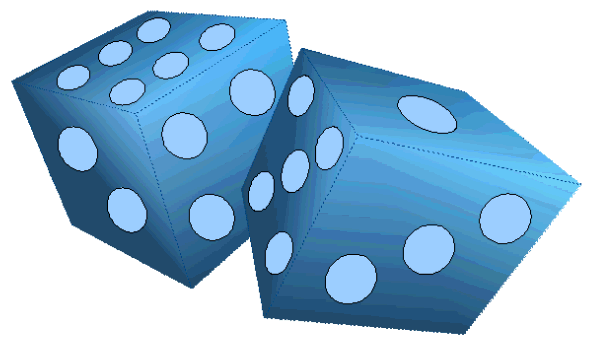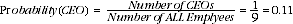1332.0.55.002 - Statistical Language!, 2008
ARCHIVED ISSUE Released at 11:30 AM (CANBERRA TIME) 27/06/2008  First Issue
Page tools:Print All
Contents >> Probability >> How do you calculate Probability?

HOW DO YOU CALCULATE PROBABILITY?

Probability is calculated by dividing the number of ways an event can occur by the total number of possible outcomes.For instance, the chance of rolling two 'ones' on a pair of dice is once out of 36 different combinations or about 0.06, to roll the sum of seven would be about 0.17, and the probability of rolling the sum of 13 on a pair of dice would be 0.Example

To calculate a probability using a working example, let's look at ‘The Widget Company’. If we put all the employees names into a hat what is the chance that we'll pull out the name of the CEO?

 Employment details of 'The Widget Company'Observation Position in company1 CEO2 Manager3 Manager4 Factory Worker5 Factory Worker6 Factory Worker7 Factory Worker8 Trainee9 TraineeWe can see that there are nine employees in total and only one CEO.Therefore, there is a one in nine chance of randomly picking the CEO out of the hat. As we said before we can also express this degree of likelihood as a number between one and zero, in this case 0.11.

 Previous Page Next Page